9. CO-ORDINATION COMPOUNDS

Q. 83. Write the formula for the following co ordination compounds :

(i) Tetra-amine diaqua cobalt (III) chloride.
(ii) Potassium tetracyanonicklate (II).
(iii) Amine bromidochloride a nitrito-N-planinate (II)
(iv) Iron (III) hexacyanoferrate (II).

Ans⇒ (i) Tetra amine diaqua cobalt (III) chloride [Co(NH3)4(H2O)2]Cl3.
(ii) Potassium tetracyanonickelate (II) -K2[Ni(CN)4]
(iii) Amine bromido chlorido nitrito-N-platinate (II)- Pt(NH3) BrCl(NO2)]
(iv) Iron (III) hexacyano ferrate (II) Fe4[Fe(CN)6l3+

Q. 84. Write the IUPAC names of the following co-ordination compounds :

(a) (CO(NH3)6]Cl3,
(b) (CO(NH3]5 Cl]Cl2,
(c) K3[Fe(CN)6],
(d) K3 [Fe(C2O4),
(e) K2[PdCl4],
(f) [Pt(NH3)2Cl(NH2CH3)]C1.

Ans⇒ (a) Hexa-amino cobalt (III) chloride.
(b) Penta amine chlorido cobalt (III) chloride.
(c) Potassium hexacyano ferrate (III).
(d) Potassium trioxalate ferrate (III).
(f) Diammine chlorido (methylamine) platinum (II) chloride.

Q. 85. Indicate the type of isomerism exhibited by the following complexes and draw structures for these isomers :

(i) K[Cr(H2O)2(C2O4)2],

(ii) [Co(en)3Cl3],

(iii) [CO(NH3)5(NO2)](NO3)2,

(iv) [Pt(NH3) H2O)(Cl2]

Ans⇒ (i) Both geometrical (cis, trans) and optical isomers for Cis can exist.
(ii) Two optical isomers can exist.
(iii) There are 10 possible isomers. There are geometrical, ionisation and linkage isomers possible.
(iv) Geometrical (Cis. trans) isomers can exist.

Q. 86. Explain Co(NH3)6]3+ is an inner orbital complex whereas [Ni(NH3)6]2+ is an outer orbital complex.

Ans⇒ In the presence of NH3, the 3d electrons pair up leaving two dorbitals empty to be involved in d2sp3. hybridisation forming inner orbital complex in case of [CO(NH3)63+

In [Ni(NH3)6]3-, Ni is in +2 oxidation state and has d8 configuration, hybridisation involved in sp3d2 forming outer obrital complex.

Q. 87. Write the formulas for the following co ordination compounds:

(i) Tetra amino aqua chlorocobalt (HI) chloride.
(ii) Potassium tetrahydroxozincate (II).
(iii) Potassium trioxalato aluminate (III).
(iv) Dichloro bis (ethane-1, 2-diamine cobalt(III).
(v) Tetracarbonyl nickel (O).

Ans⇒ (i) (CO(NH3)4 (H2O)Cl]Cl2
(ii) K2[Zn(OH)4]
(iii) K3[Al(C2O4)3]
(iv) (CoCl2(en)]+
(v) [Ni(CO)4).

Q. 88. Write the IUPAC names of the following Co-ordination compounds :

(i) [Pt(NH3)2Cl(NO2)] (ii) K3[Cr(C2O4)3]
(iii) (CoCl2(en)2]Cl
(iv) [Co(NH3)6(CO3)C1
(v) Hg(Co(SCN)4].

Ans⇒ (i) Diamine chloronitrito-N-platinum (II).

(ii) Potassium trioxalato chromate (III).
(iii) Dichloro bis ethane-1, 2-diamine cobalt (III) chloride.
(iv) Hexa amine carbonato cobalt (III) chloride.
(v) Mercury tetrathiocyanato cobaltate (III).

Q. 89. Explain the bonding in co-ordination compounds in terms of Werner’s postulates.

Ans⇒ Postulates are :

(i) In co-ordination compounds metals show two types of linkages (valencies)-parimary and secondary.

(ii) The primary valencies are normally ionisable and are satisfied by negative ions.

(iii) The secondary valencies are non-ionisable. These are satisfied by neutral molecules or negative ions. The secondary valence is equal to the coordination number and is fixed for a neutral.

(iv) The ions/ groups bound by the secondary linkages to the metal have characteristics spatial arrangements corresponding to different numbers.

In the modern formulation, such spatial arrangements are called co-ordination entities or complexes and the ions out side the square bracket are called counter ions.

He further postulated that octahedral, tetrahedral and square planar geometrical shapes are more common in co-ordination compounds of transition metals. Thus

[Co(NH3]6]3+, [COCl(NH3)52+ and [CoCl2 (NH3)4+ are octahedral while (Ni(Co)4] and (PtCl4]2- are tetrahedral and square planar respectively.

Q. 90. Using IUPAC norms write the formulae of the following:

(i) Hexa amine cobalt (III) sulphate.
(iii) Potassium tri (oxalato) chromate (III).
(iv) Dimine dichloro platinum (II).
(v) Hexa amine platinum (IV).
(vi) Potassium tetracyano nickelate (II).

Ans⇒ (i) (CO(NH3]2(SO4)3

(ii) K2[PdCl4]
(iii) K3[Cr (C2O4)3]
(iv) [Pt(NH3)2Cl2]
(v) [Pt(NH3)64+]
(vi) K2[Ni(CN)4]

Q. 91. Using IUPAC norms write the systematic name of the following:

(i) (Co(NH3)6Cl3
(ii) [Co(NH3)4 Cl(NO2)]Cl
(iii) [Ni(NH3)6]Cl2
(iv) [Mn(H2O)62+
(v) [Co(en)3]3+
(vi) [Ti(H2O)6]3+
(vii) [NiCl4]2+
(viii) [Ni(Co)4]

Ans⇒ (i) Hexa-amino cobalt (III) chloride.
(ii) Tetra-amino chloro nitro cobalt (III) chloride.
(iii) Hexammine nickel (II) chloride.
(iv) Hexa-aqua manganese (II) ion.
(v) Tris (1, 2-ethane diamine) cobalt (III) ion.
(vi) Hexa-aqua titanium (III) ion.
(vii) Tetrachloronickelate (II) ion.
(viii) Tetra cabonyl nickel (O).

Q. 92. Draw the structures of optical isomers of

(i) [Cr(C2O4)3]3-

(ii) [PtCl2 (en2)]2+

(iii) (Cr(NH3)2Cl2 (en)]+

Ans⇒ (i) The two optical isomers of (Cr(C2O4)3]3- are C2O24 is in short ox :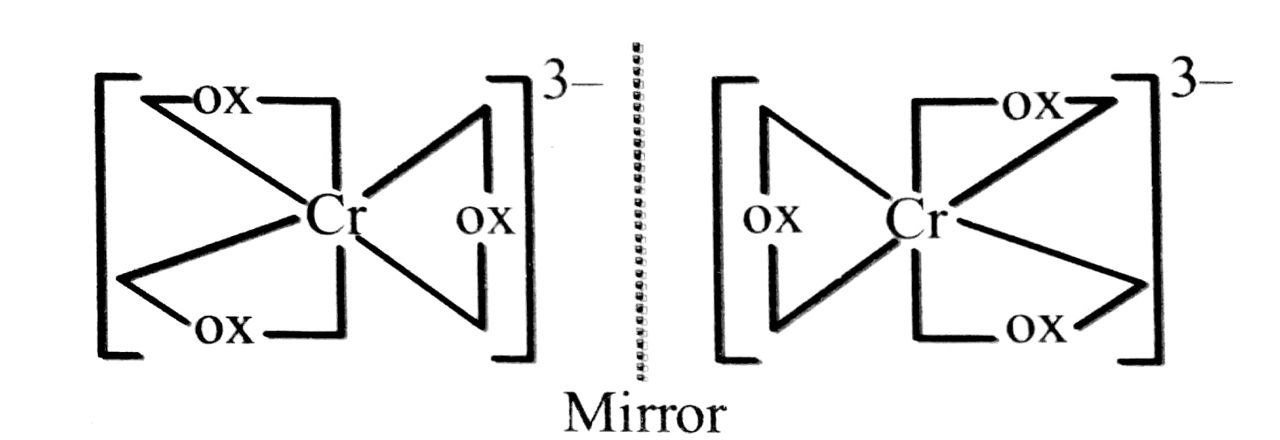Non-superimposable mirror image on the objec
(ii) The two optical isomers of [PtCl2 (en)2]2+ are: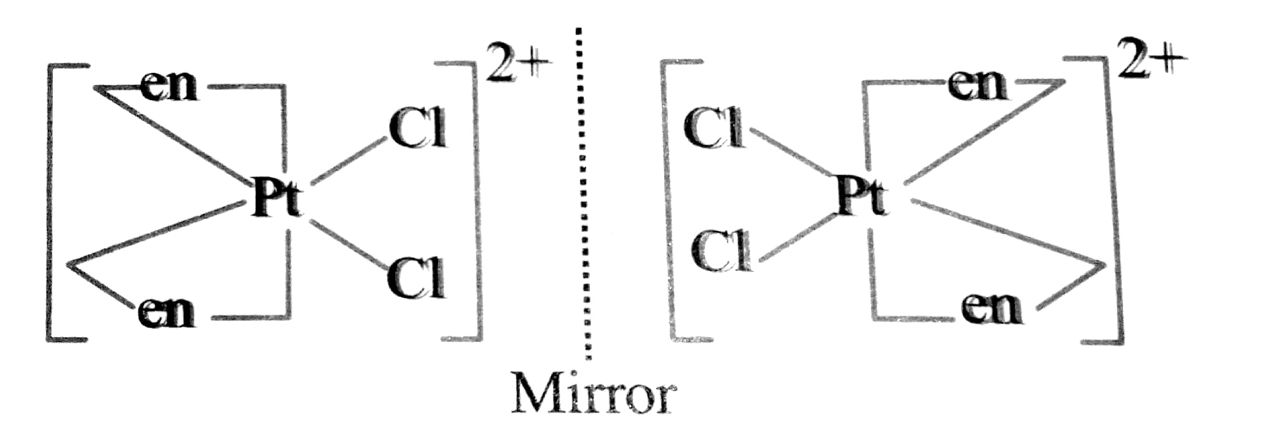(iii) [Cr(NH3)2Cl2 (en)]+: The two optical isomers are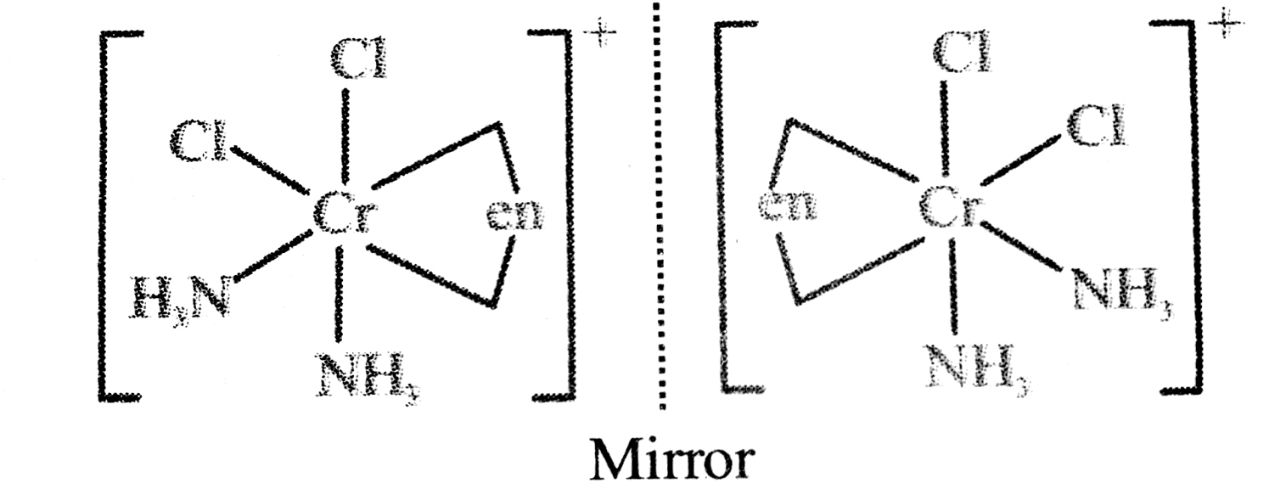Q. 93. Draw all the isomers (geometrical and optical) of

(i) (CoCl2 (en)2]+
(ii) (Co(NH3)Cl(en2)2+
(iii) Co(NH3)2 Cl2 (en)]+

Ans⇒ (i) (CoCl2 (en)2]+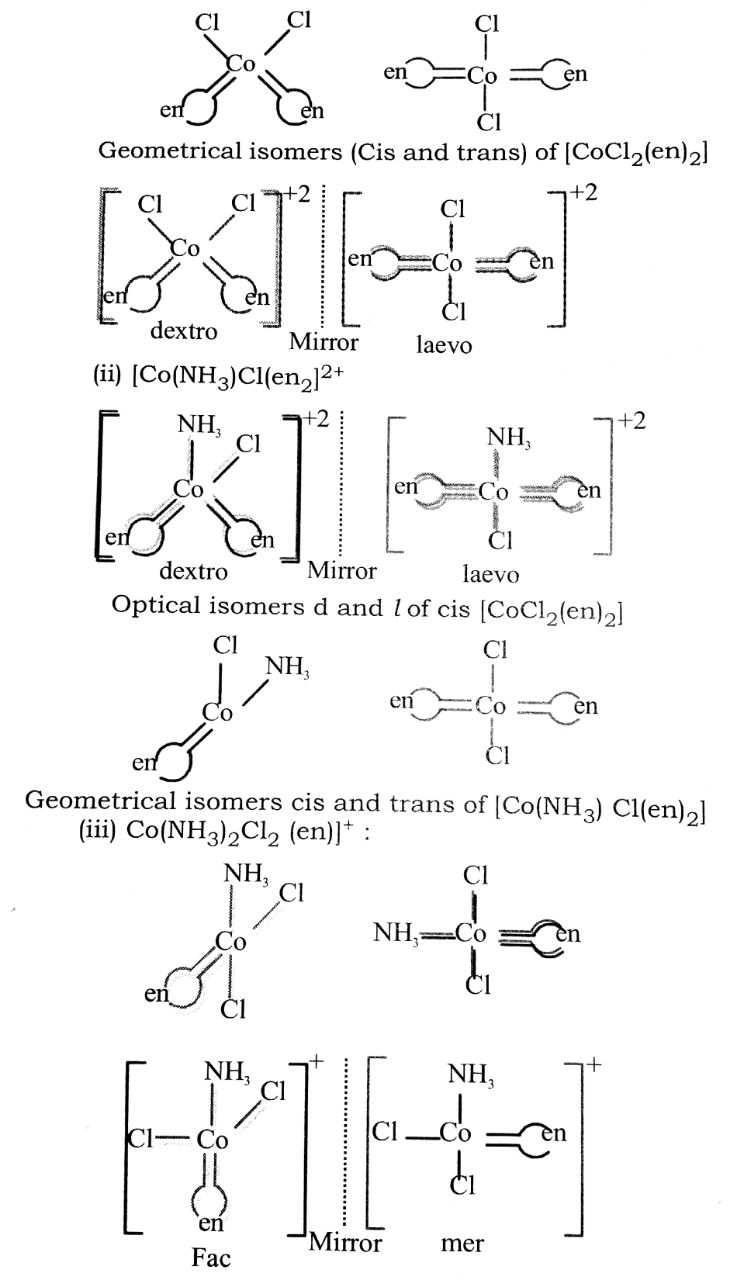Q. 94. Discuss the nature of bonding in the following co-ordination entities on the basis of valence bond theory.

(i) [Fe(CN6)4-
(ii) (FeF6]3-
(iii) (Co(OX)3]3-
(iv) (CoF6]3-

Ans⇒ (i) [Fe(CN6]4-

Outer electronic configuration of iron (Z = 26) in ground state is 3d64s2. Iron in this complex is in + 2 oxidation state. Iron achieves + 2 oxidation state by the loss of two 4s electrons. The resulting Fe2+ ion has outer electronic configuration of 3d6.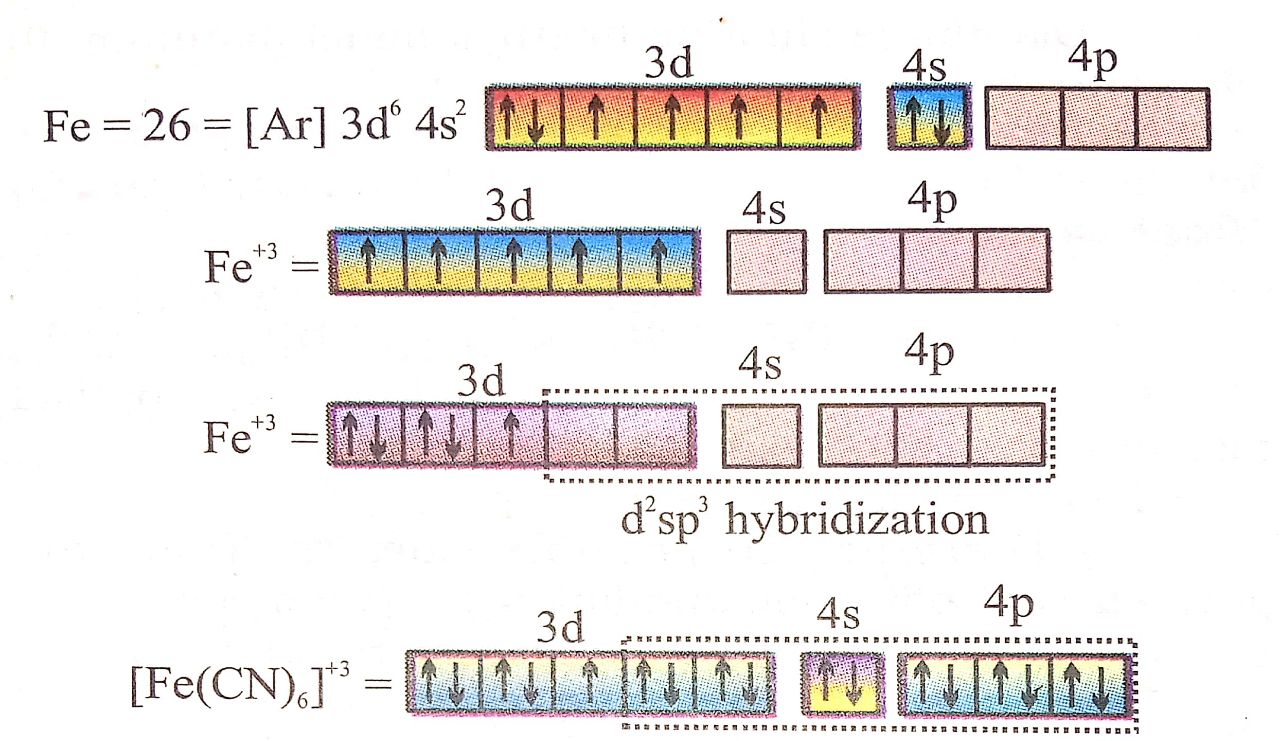It has been experimentally observed that this complex is diamagnetic as such has no unpaired electron. To account for this two unpaird electron in the 3d-subshell pair up, this leaving two 3d-orbitals empty. These two vacant 3d-orbitals along with one 4s-orbital and three 4p-orbitals hybridise to give six equivalent d2sp3 hybridised orbitals. Six pairs of electrons one from each cyanide ion, occupy the six vacant hybrid orbital so produced. The resulting complex ion has an octahedral geometry and is diamagnetic due to the absence of unpaired electrons.

(ii) Bonding in [FeF6]3-

The oxidation state of Fe is + 3 and its co-ordination number is 6. The complex has octahedral geometry and experimental study shows that it is paramagnetic.

The bonding in this entity is explained on the basis of overlap of sp3d2 hybrid orbitals of Fe3+ ion and six ione pair orbitals of cyanide ligands. Five 3d-electrons are unpaired which make it paramagnetic. The outer electronic configuration of Fe3+ ion is 3d5 which is highly stable and no pairing of electrons takes place even in presence of strong field of F-ions. Here, 4d orbitals of Fe3+ (which are empty) are involved.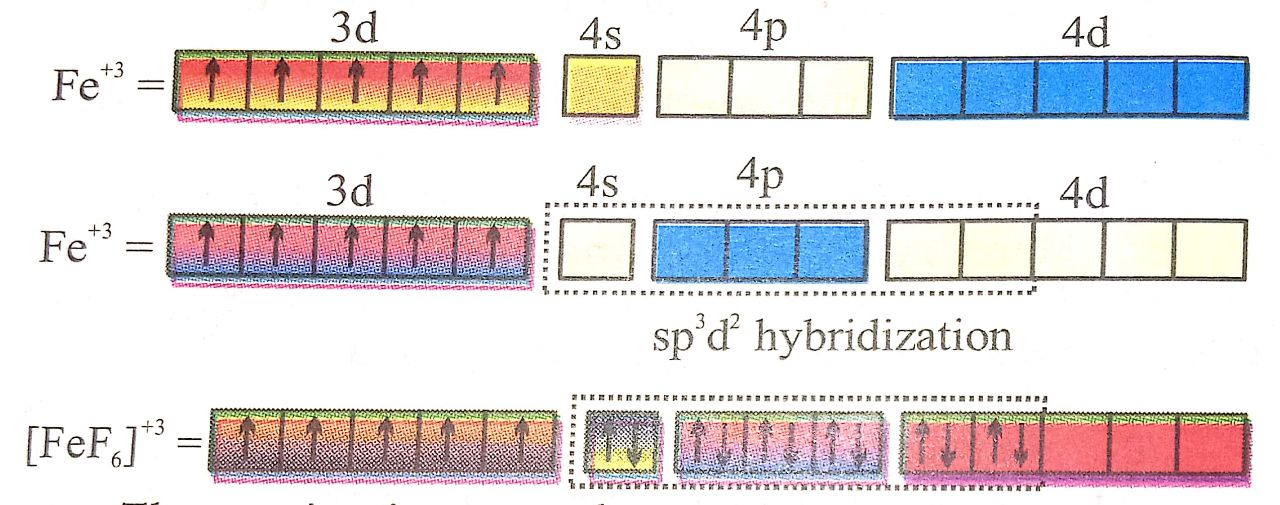The entity is strongly paramagnetic due to five unpaired electrons and is an outer orbital complex.

(iii) Bonding in (Co(OX)3]3-
As didenate oxalate ions approach of pairing of electrons in 3d-orbitals takes place.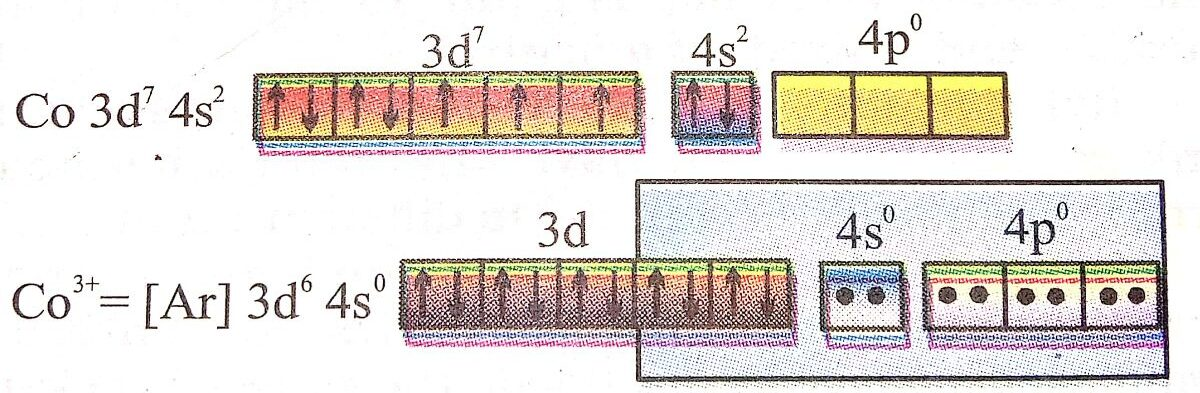Because all electrons are paired.  Hence diamagnetic.

(iv) [CoF6]3- oxidation state of Co is +3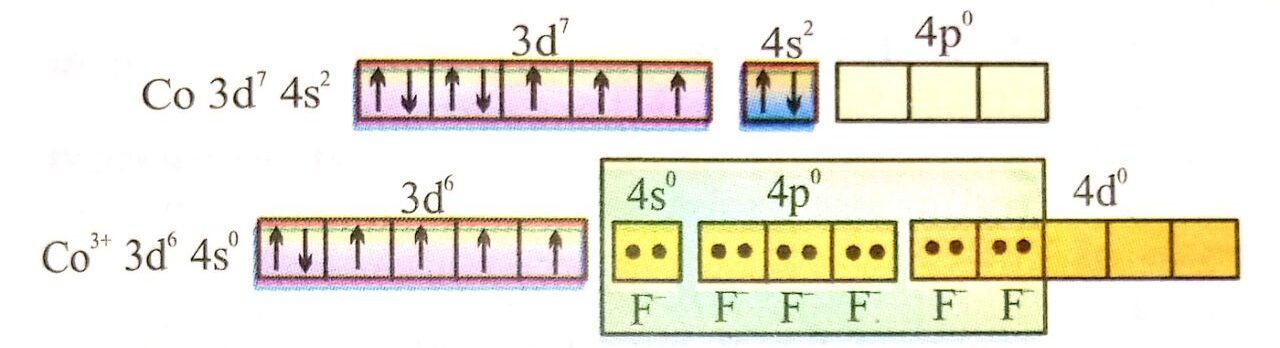As there are four unpaired electrons, the complex is paramagnetic in nature.

Q. 95. Discuss briefly the role of co-ordination compounds in

(a) biological systems and (b) analytical chemistry (c) medicinal chemistry and extraction/ metallurgy of metals.

Ans⇒ (a) Biological system :

(i) Haemoglobin the red blood cell which acts as oxygen carrier to different parts of the body is a complex of iron (II).
(ii) Vitamin B12 is a complex of cobalt metal. Trace metals also function through co-ordination processes.
(iii) A compelx of zinc (II) called enzyme CPA helps in digestion of food.
(iv) The chlorophyll, the green colouring plant pigment that plays an important role in photosynthesis is a complex of magnesium. In chlorophyll Mg is co ordinated to four nitrogens of porphyin units.

(b) Analytical chemistry :

(i) Multidentate ligand, EDTA (ethylene diamine tetra acetic acid) forms highly stable complexes with metal ions like Ca2+ and Mg2+. This fact is used to estimate the hardness of water by simple titration method using EDTA solution.

(ii) A confirmatory test for the detection of copper (II) involves the formation of a deep-blue coloured complex, [Cu(NH3)4]2+. on addition of ammoniasolution to asolution of copper (II) salt.

Cu2+ + 4NH3+ → [Cu(NH3)42+

Tetra amine copper (II) ion (Deep blue)

(iii) The separation of group 1 precipitate of AgCl2 HgCl2 and PbCl2 involves in addition of aqueous ammonia solution to the precipitate, when only silver chloride dissolves, due to the formation of the complex ion. (Ag (NH3)2]+

AgCl + 2NH3 → [Ag(NH3)2]+ + Cl

Hg2 Cl2 and PbCl2 do not form complex ions with NH3 and hence, do not dissolve.

(iv) A confirmatory test for nickel consists in addition a solution of dimethyl glyoxime, when a scarlet-red coloured precipitate is formed due to the formation of a chelate complex.

(c) Medicinal Chemistry : compounds are used in medicines. Some common examples are :
(i) Vitamin B12 used to prevent anaemia is a complex compound of cobalt.
(ii) The complex of calcium and EDTA is used a the treatment of lead poisoning. Inside the body calcium in e complex is replaced by lead. The more soluble lead EDTA complex is eliminated is urine.
(iii) The platinum complex cis-[Pt(NH3)2 C12] known as cis platin is used in the treatment of cancer.

(d) Extraction/metallurgy of Metals :

(i) Extraction metals like silver and gold is carried out by forming their soluble cynide complex, e.g.,

4Au + 16Cn + 6H2O + O2 → 4[Au(CN)2] 12OH

Dicyano gold (I) (soluble)

The solution containing cyanide complex is then treated with zinc, when gold is precipitated.

2[Au(CN)2]Zn → [Zn(CN)4]2- + 2Au

(ii) Co-ordination compounds of silver and gold are used as the constituents of electroplationg baths for the con trolled delivery of Ag+ and au ions, during electro refining of these metals.

Q. 96. Give the principle of Habber’s process for the manufacture of NH3. HOW NH3 is converted into HNO3 Write principle and equation only.

Ans⇒ Habber’s process: Ammonia is manufactured by direct combination of nitrogen and hydrogen at very high pressure (about 200 atm) and low temp (450’c) in presence of catalyst.

N2 + 3H2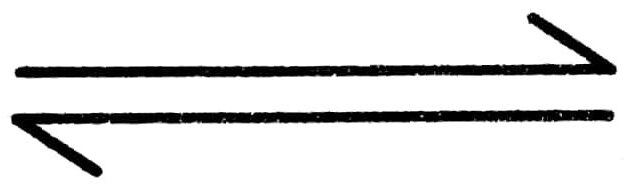2NH3 + QKCal

The reaction is reversible, exothermic and there is contraction in volume. Therefore, maximum yield of ammonia will be at high pressure, low temperature and removal of ammonia from the system to make only forward reaction.

Ammonia is converted to nitric acid by catalytic oxidation of ammonia in presence of platinised asbestas to get nitric oxide.

4NH3 + 502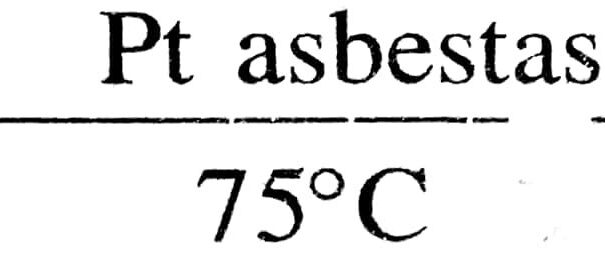→ 4NO + 6H2O

Nitric oxide with excess of oxygen is changed into NO2 and then into HNO3

2NO + O2 – → 2NO2

3NO2 + H2O – → 2HNO3 + NO

Q. 97. Arrange the following in the order of property indicated for each set :

(a) Fe2, Cl2, Br2, I2 – Increasing order of bond energy.
(b) NH3, PH3, AsH3, SbH3, BiH3 – Increasing base strength.
(c) HOCI, HOBr, HOI – Increasing acid strength
(d) H2O, H2S, NH3, XeF2 – Increasing bond angle
(e) HF, HCI, HBr, HI-Increasing thermal stability

Ans⇒ (a) I2 < Br2 <F2 < Cl2
(b) BiH3 SbH3 < ANH3 < PH3 < NH3
(c) HOI < HOBr < HOCl
(d) H2S < H2O < NH3 < XeF2
(e) HI < HBr < HCl < HF

Q. 98. Account for the following:

(a) Sulphur in the vapour state exhibits paramagnetism.
(b) Noble gases form compounds with fluorine and oxygen only.
(c) Bleaching of flowers by chlorine is permanent while that by sulphur dioxide is temporary why ?

Ans⇒ (a) In vapour State (~1000k). Sulphur partly exists as S2 molecule like O2 has two unpaired electrons in the anti-bonding i molecular orbital and exhibits Para magnetism.

(b) It is because F2 and O2 are the best oxidizing agents
(c) In presence of moisture, chlorine acts as an oxidizing agent and a bleaching agent

Cl2 + H2O → HCl + HOCI
.                             Unstable

HOCl → HCl + O Nascent oxygen.

Coloured mater + 0 → Colourless matter.

The bleaching action is permanent due to nascent oxygen. Bleaching action of SO2 in presence of moisture is due to nascent hydrogen.

2SO2 + 2H2O → H2SO4 + 2H

Coloured matter + H → colourless matter.
Therefore bleaching action of SO2 is temporary.

Q. 99. Account for the following :

(a) HI is sponger acid than HF.
(b) The electron affinity of fluorine is less than that of chlorine.
(c) Formic acid is stronger than Acetic acid.
(d) Aniline is less basic than methylamine.
(e) PCl5 is known but NCl5 is not known.
(f) Fluorine shows only one oxidation states but rest of the halogeus show different oxidation states.
(g) H2O is a liquid but H2S is gas.
(h) Noble gase are monoatomic in nature.
(i) Bleaching of flowers by chlorine is permanent but that by sulpher dioxide is temporary.
(j) NH3 has higher S.P. than PH3.
(k) Write the structural formula of XeOF4.

Ans⇒ (a) Due to bigger atomic size of iodine with respect to fluorine. HI is sponger acid than H – F.
(b) Bond dissociation energy decreases as bond distance increasing flowerr. The F – F bond disociation energy is smaller than that of Cl – Cl. Due to this the electron affinity of fluorine to less than that of chlorine.
(c) Acidity of an acid mainly depends upon release of proton from the solution.
(d) One lone pair of electron is present on Nitrogen atom in Aniline whichis over lodded with -electron is the ring. Hence, Nipogen atom is + only charged and not in the shape that if can give methyl amine.Hence aniline is less basic than methyl amine.
(e) Due to last of d-sub-shell Nitrogen cannot from NC15.
(f) Due to lack of d-subshell fluorine shows only one oxidation state. While rest shows different oxidation state.
(g) Due to Hydrogen bonding water is liquid, but H2S is gas.
(h) Noble gases are non-atomic, bond oder is found in this case zero.
(i) Chlorine shows bleching action, through orxygen which to permanent.
But SO2 shows bleaching action through Hydrogen which is temporary.
(j) NH3 is associated molecule due to H-bonding and so molecular mass is high and as such it is liquid which PH3 is monomeric law mol. mas and hence a gas.
(k) Of the noble only xeron from chemical compounds. Xe has comparable I.P. than oxidised cation so Xenon from compounds with highly electron element fluorine.

Q. 100. I. Arrange following in the increaing properties :

(a) HF, HC1, HBr, HI (acidic strength)

(b) H2O, H2S, H2Se, H2Te (B.P.)

(c) F2, Cl2, Br2, I2 (Bond dissociation energy)
II. Predict the probable structure of BrF3 on the basis of VSEPR theory.

Ans⇒ I. (a) HF < HCl < HBr < HI
(b) H2S <H2Sc <H2Tc < H2O
(c) I2 < F2 < Br2 < Cl2

II. The central atom Br has 7 Valence Electron. Three of these will form covalent bond with three F-atom leaving behind for electrons. Thus there are three bond pairs and two lone pairs. According to VSEPR — Theory, these bonds will occupy the corners of a trigonal bipyramid two lone pairs will occupy equatorial position to minimise lone pair – lone pair and B.P.-L.P. repulsion, which are greater than B.P. – B.P. repulsion. In addition, the axial F-atom will be bent towards the equatorial F in order to minimise the L.P. – L.P. repulsion Therefore shape would be slightly bent T.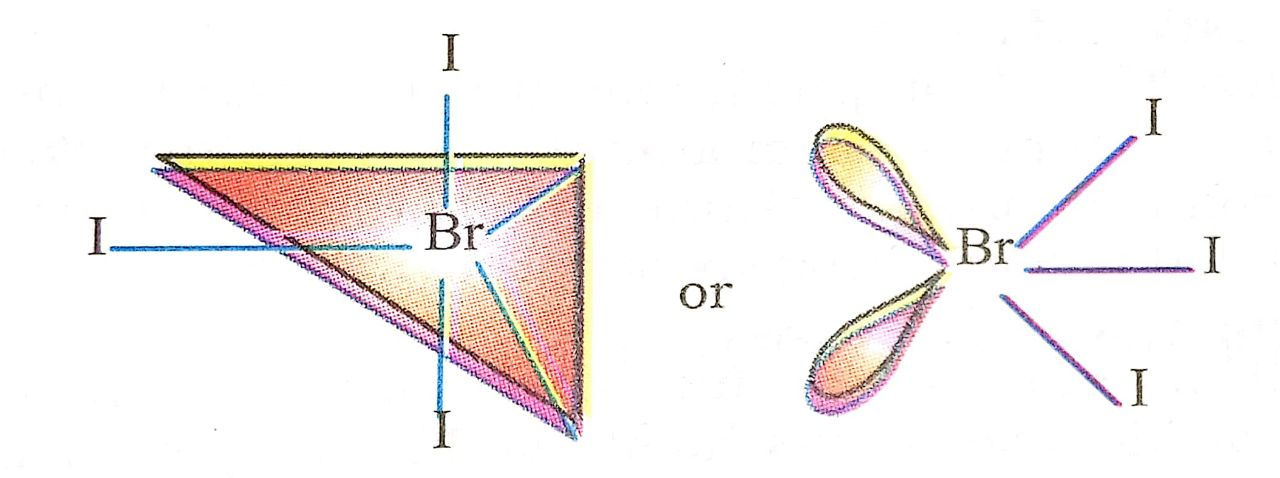Q. 101. (i) Write the electronic configuration of Cu2+, Z = 29 for Cu.
(ii) Discuss the oxidation states of lanthanides.
(iii) What is the trend in the ionic radii of transition elements ?
(iv) Account for the fact that the second ionisation energies of both chromium and copper are higher than those of the next element.
(V) Why is manganese more stable in the +2 state than the +3 state while the reverse is true for iron ?

Ans⇒ (i) Cu atom contains 29 electrons but Cu2+ ion contains 27 electrons. These electrons are arranged as
Cu2+ = ls2 2s2 2p6 3s2 3p6 3d9.

(ii) Typical oxidation state of all lanthanides is + 3. Some lanthanides also exhibit +2 and +4 oxidation states in addition to + 3. For example cerium forms salt in +4 oxidation state Europium (II) salts are also known. These additional oxidation states are possible only because of the extrastability of an empty half-filled or completely filled orbitals in some lanthanides. For example, in Ce4+ f-orbital is empty in Eu2+ and Yb2+ f-orbital is completely filled (4f14 5d06s0).

(iii) The ionic radii decrease with the increase in the atomic numbers of transition elements in horizontal row. The decreasing trend is attributed to the increasing pull on the d-electrons by the nucleus.

(iv) In chromium and copper the second electron is lost from (n − 1) d-orbitals, whereas in the next higher element it is from ns.

(v) Mn2+ and Fe3+ have half-filled 3d-orbitals that makes them more stable than Mn3+ and Fe2+ respectively.

Q. 102. Illustrate with an example each of the following :

(a) Ionization isomerism
(c) Co-ordination isomerism.

Ans⇒ (a) Ionization isomerism : The compounds which have same molecular formula but give different ions in solution are called ionisation isomer. In this type of isomerism, the difference arises from the interchange of groups within or outside the co-ordination spheres. Therefore, these isomers give different ions in solution. For example :

[Co(NH3)5 Br]SO4                     [Co(NH3)5 SO4 ]Br

Bromo penta-amine cobalt (III) Suphate penta-amine cobalt
suphate (violet in colour) (III) bromide (red in colour)

(b) Linkage isomerism : The compounds which have the same molecular formula but differ in the mode of attachment of a ligand to the metal atom or ion are called linkage isomers. For example, in NO2 ion, the nitrogen atom as well as the oxygen atom can donate their lone pairs. This gives rise to isomerism. If nitrogen donates its lone pair, one particular compound will be formed. On the other hand, if oxygen donates its lone pair, a different compound is obtained. If the bonding is through N, the ligand is named as nitro and if it is through O, it is named as nitrito.

NO2nitro ONO nitroto

For example, two different penta-amine cobalt (III) chlorides each containing the NO2, group in the complex ion have been prepared. These are :

[Co(NH3)5(NO)2]Cl2 Penta-amine nitro cobalt

Yellow brown      (III) chloride

[Co(NH3)5(ONO)]Cl2 Penta-amine nitrito cobalt (III) chloride.

(c) Co-ordination isomerism : This type of isomerism occurs in compounds containing both cationic and anionic complexes and isomers differ in the distribution of ligands in the co-ordination sphere of cationic and anionic parts. The example are :

(i) [Co(NH3)6][Cr(CN)6 and [Cr(NH3)6[Co(CN)6]

(ii) [Cu(NH3)4][PtC14]and[Pt(NH3)4[CuCl4]

This type of isomerism is also shown by compounds in which the metal ion is the same in both cationic and anionic complexes. For example :

(i) [Cr(NH3)6 ][Cr(CN)6] and

[Cr(NH3)4 (CN)4[Cr(NH3)2 (CN)4]

(ii) [Pt(NH3)4][PtCl4] and [PtCl(NH3)3[PtCl3(NH3)].

Q. 103. Explain the bonding in coordination compounds in terms of Werner’s postulates

Ans⇒ Werner’s Postulates for bonding in coordination compounds :

1. Every metal atom possesses two types of valencies (or linkages).

2. (a) Primary linkage corresponds to the oxidation state of the metal atom.
(C) Primary linkages are satisfied with negative ions.

3. (a) Secondary linkage corresponds to the coordination number of the metal atom.
(b) Secondary linkage are non-ionisable. The coordination number of a metal is a fixed number
(c) Secondary linkages are satisfied either by negative ions or neutral ligands.
(d) Secondary linkages are directed towards fixed positions in space about the central metal atom or ion.

As a result, the coordinaion compound acquires a definite shape or geometry.

In the light of the postulates above, Werner, who had prepared the following compounds by reacting Cobalt (III) chloride with NH3, successfully explained the structure of these coordination compounds.

CoCl3. 6 NH3                        Organe yellow

CoCl3. 5NH3. H2O        Pink
CoCl3. 5NH3.                Violet
CoCl3. 4NH3.                Violet }
COCl3. 4NH3.               Green}

Different colours of CoCl3. 4NH3 compound is due to the fact that they are cis and trans isomers.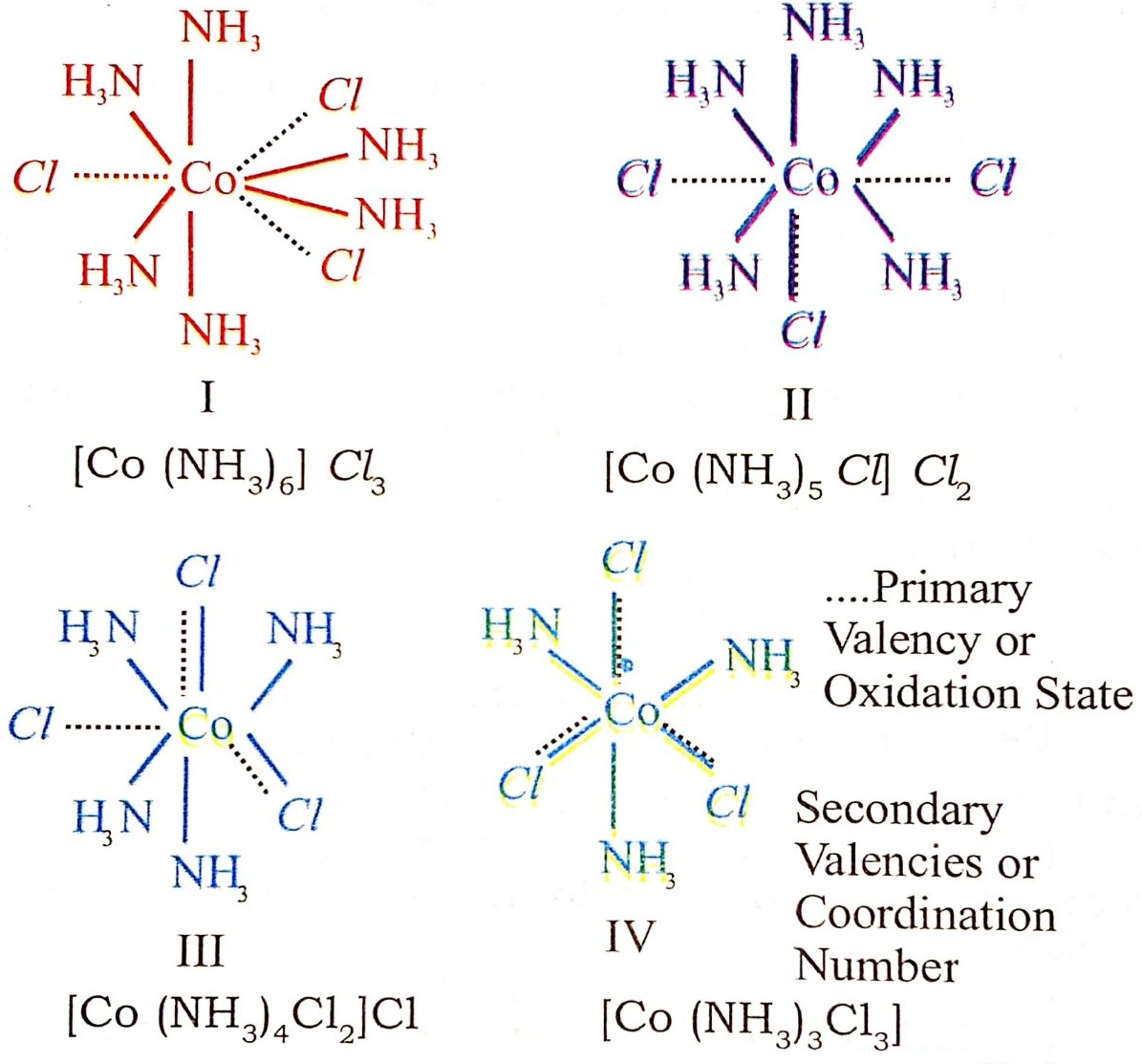Q. 104. What is meant by stability of a coordination compound in solution ? State the factor which govern stability of complexes.

Ans⇒ The stability of a complex in solution refers to the degree of association between the two species involved in the state of equilibrium. The magnitude of the (stability or formation) equilibrium constant for the association; quantitatively expresses the stability. thus, if we have a reaction of the type.

M + 4 L → ML4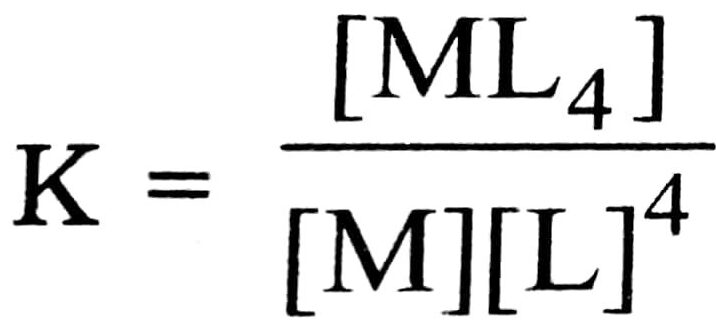Larger the stability constant, the thigher the proportion of ML4 that exists in solution.

Factors on which stability of the complex depends:

(i) Charge on the central metal ion : Greater the charge on the central metal ion, greater is the stability of the complex.

(ii) Nature of the metal ion : Groups 3 to 6 and inner transition elements form stable complexes when donor atoms of the ligands are N, O and F. The elements after group 6 of the transition metals which have relatively d-orbitals (e.g., Rh, Pd, Ag, Au, Hg etc.) form stable complexes when the donor atoms of the ligands are heavier members of N, O and F family.

(iii) Basic nature of the ligand : Greater the basic strength of the ligand, greater is the sability of the complex.

(iv) Presence of chelate rings in the complex increases its stability. It is called chelate effect. It is maximum for the 5- and 6-membered rings.

(v) Effect of multidentate cyclic ligands : If the ligands happen to be multidentate and cyclic without any steric effect, the stability of the complex is further increased.

## Class 12th Chemistry Long Type Question

 S.N CHEMISTRY LONG TYPE QUESTION 2022 1 SOLID STATE 2 SOLUTION 3 ELECTROCHEMISTRY 4 CHEMICAL KINETICS 5 SURFACE CHEMISTRY 6 GENERAL PRINCIPLES AND PROCESSES OF ISOLATION OF ELEMENTS 7 THE P-BLOCK ELEMENTS 8 THE D- AND f-BLOCK ELEMENTS 9 CO-ORDINATION COMPOUNDS 10 HALOALKANES AND HALOARENES 11 ALCOHOLS, PHENOLS AND ETHERS 12 ALDEHYDES, KETONES AND CARBOXYLIC ACIDS 13 AMINES 14 BIOMOLECULES 15 POLYMERS 16 CHEMISTRY IN EVERYDAY LIFE 17 DISTINGUISH BETWEEN PAIR 18 CONVERSIONS